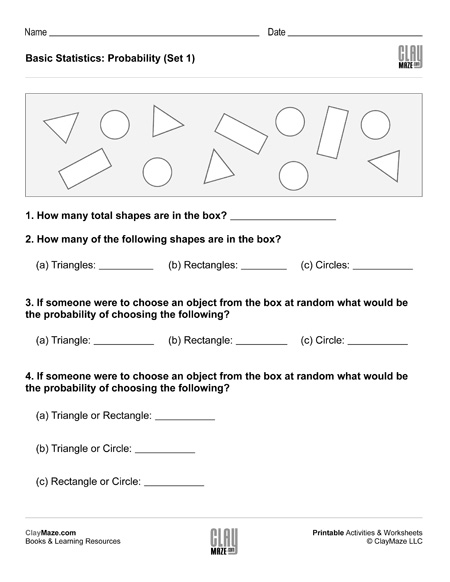Basic Statistics: Probability I (Set 1)

This statistics worksheet is on basic probability. There are a number of different shapes in the box and the student is asked to find the probabilities of selecting the different shapes at random.